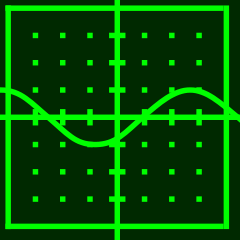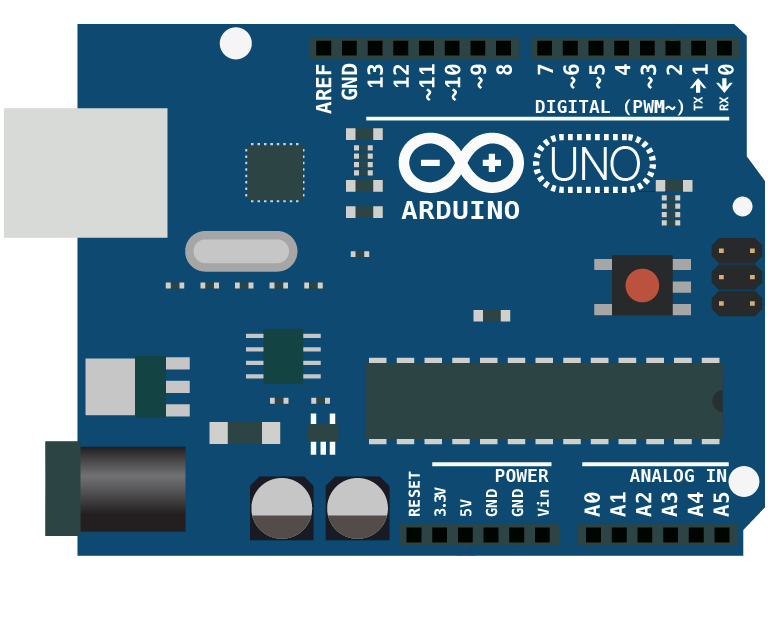# Chris Greenley## PHYS3098: Circuits

April 4, 2017

Teaching circuits to undergrads. From Ohm’s law to opamps and microcontrollers.

I taught the circuits lab for physics majors three times, the first time as a grad student and the second and third times as an instructor. I really enjoyed teaching this lab, partly because I learned so much myself.

The second year, I did a complete rewrite of all the labs. Below are links to the full labs and summaries of what was covered in each lab.

#### Lab 1 - Instrumentation and Measurements (pdf)Using a multimeter without letting the magic smoke out of the fuse, operating a variable DC power supply and a function generator, building circuits on breadboards, voltage divider circuits, and proper oscilloscope usage. Ends with a discussion of uncertainty and propagation thereof.

#### Lab 2 - Input and Output Impedance (pdf)Measure the input impedance of a voltmeter and an oscilloscope, and observe the effects of measurement equipment loading a circuit. Analyze resistive and capacitive voltage dividers. Measure the output impedance of a function generator.

#### Lab 3 - RC Circuits (pdf)Measure the time constant of charging and discharging RC circuits. Derive RC circuit voltages using complex analysis and use this to build integrator and differentiator circuits with outputs accurate to within 1%. I created a YouTube video showing an example derivation.

#### Lab 4 - Thevenin Equivalence and Filters (pdf)Analyze a resistor circuit using Thevenin’s Theorem, construct low and high pass RC filters, create Bode plots from amplitude data, build LC bandpass and notch filters and use an oscilloscope as a simple spectrum analyzer.

#### Lab 5 - Wave Behavior and Filter Design (pdf)Use a bandpass filter to pull out the Fourier components of a square wave. Design and construct a two stage RC and a third order Butterworth low pass filter.

#### Lab 6 - Filters, Diodes, and Power Supplies (pdf)Combine three sine waves of different frequencies and design three filters to pull each one back out. Compare small signal, Schottky and Zener in a half wave rectifier. Build a variable regulated DC power supply.

#### Lab 7 - LTSpice Simulations and IV Curves (pdf)Use LTSpice to simulate a voltage divider, a high pass filter in the time domain, create a Bode plot, and the IV curve for a diode. Measure the real life current and voltage values for a diode and create an IV plot.

#### Lab 8 - Transistors I (pdf)Measure collector and base current and base-emitter voltage for NPN and PNP transistors. Create plots of collector current as a function of base-emitter voltage, and collector current as a function of base current. Design and construct a “night light” circuit which turns on an LED when the ambient lights gets dim.

#### Lab 9 - Transistors II (pdf)Construct an unbiased emitter follower. Design and construct a biased emitter follower. Construct an LC oscillator and connect to the biased emitter follow to demonstrate its use as a buffer amplifier and impedance transformer. Use the oscilloscope to build a curve tracer and examine the curves for diodes, resistors, capacitors, inductors and transistors.

#### Lab 10 - Operational Amplifiers (pdf)Construct an inverting amplifier and a summing amplifier. Build a closed loop PID constant illumination controller using eight opamps and a phototransistor.

#### Lab 11 - Digital Logic and Audio Synths (pdf)Construct NAND, NOT, AND, OR and NOR gates using NAND logic with LEDs showing the state of each gate. Using 555 timer ICs construct astable and monostable multivibrators. Link these together to form a simple audio synthesizer, often called the Atari Punk Console.

#### Lab 12 - Microcontrollers I(pdf)Set up the Arduino IDE and upload code to a SparkFun Redboard. Use the microcontroller to control an RGB LED. Read in values from a flex sensor and set the position of a servo accordingly. Control a relay via a transistor and use a scope to show why a flyback diode is needed. Display text on an LCD.

#### Lab 13 - Microcontrollers II(pdf,code)Decode the signal from an IR remote. Build a 5-bit R-2R digital to analog converter (DAC). Hook up the DAC to a common collector amplifier and a speaker. Synthesize sine waves corresponding to musical notes using direct digital synthesis (DDS). Use the remote control to select various frequencies, creating a remote controlled piano.